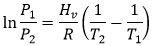What Is Meant By Vapour Pressure? Relation between Vapour Pressure and Temperature

What is vapour pressure?

Consider vaporization of a liquid (for example we take water) or solid in a closed vessel. At equilibrium the amount of water evaporating equal to amount of water condensing ( i.e. no more evaporation of water). Then the vapour pressure is defined as the pressure exerted by the vapour on the surface of liquid at in equilibrium condition. The equilibrium vapour pressure is a relative measure of liquid's evaporation rate. The substance with high vapour pressure at normal temperature is considered as highly volatile.

Vapour pressure of a liquid varies with its temperature. Vapour pressure increases with increasing temperature or vapour pressure decreases as the temperature decreases. When temperature increases the kinetic energy of liquid increase and number molecules transition into vapour increases, thereby increasing the vapour pressure.

Clausius-Clapeyron relation to find vapour pressure

The vapour pressure of substance can be determined with the Clausius–Clapeyron relation.Here P1 and P2 are vapour pressure of material at Temperature T1 and T2
Hv = Enthalpy of vaporization of material
R = Ideal gas constant, 8.314 J/mol.K

Figure shows vapour pressure of water at various temperature.

Remember:
👉The type of container surface (glass, metal etc) that contact with liquid has no effect on vapour pressure.
👉Molecule with low intermolecular force will show higher vapour pressure than the molecule with high intermolecular forces.